# Olympiad Test: Cube And Cube Roots - 2

## 10 Questions MCQ Test Mathematical Olympiad Class 8 | Olympiad Test: Cube And Cube Roots - 2

Description
Attempt Olympiad Test: Cube And Cube Roots - 2 | 10 questions in 20 minutes | Mock test for Class 8 preparation | Free important questions MCQ to study Mathematical Olympiad Class 8 for Class 8 Exam | Download free PDF with solutions
QUESTION: 1

### Which of the following is a perfect cube?

Solution:

343 = 7 × 7 × 7
∴ 343 is a perfect cube.

QUESTION: 2

### What is the value of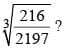Solution: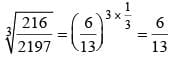QUESTION: 3

### ​The value of x3y2, if x= 3, y= -3 will be

Solution: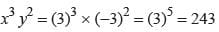QUESTION: 4

Find the least number which should be added to 500, in order to make the 500, a perfect cube.

Solution:

500 = 2 × 5 × 5 × 5 = 2 × 53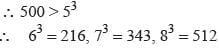∴ 12 should be added to make the sum, a perfect cube

QUESTION: 5

What is the least number which should be subtracted from 1370, in order to make the resultant, a perfect square?

Solution:

1370 =  2 × 5 × 137 1370 > 103 = 1000
∴ 113 = 1331, 123 = 1728
∴ 1370-1331 = 39
∴ Required number = 39

QUESTION: 6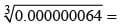Solution: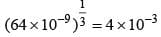= 0.004

QUESTION: 7

​193 – 93 will have – as its one of the factors

Solution: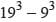will have (19 – 9) has one of its factors
∴ 10 will be a factor of (193 – 93)

QUESTION: 8

​213 + 273 will have – as its one of the factors

Solution:

21+ 27has (21 + 27) as its one of the factors
∴ 48 will be a factor of (213 + 273)

QUESTION: 9

Which is the least number which should be added to 1720,in order to make it a perfect cube?

Solution:

1720 > 1000 = 103
∴ 113 = 1331, 123 = 1728
∴ 1728 – 1720 = 8 should be added to 1720 to make the sum, a perfect cube.

QUESTION: 10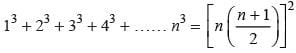where ‘n’ is a natural number, then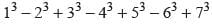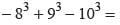Solution: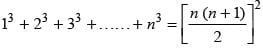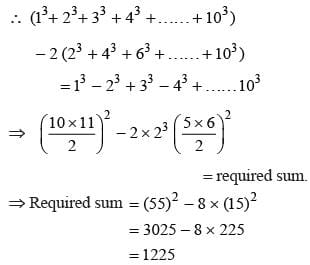Use Code STAYHOME200 and get INR 200 additional OFF Use Coupon Code